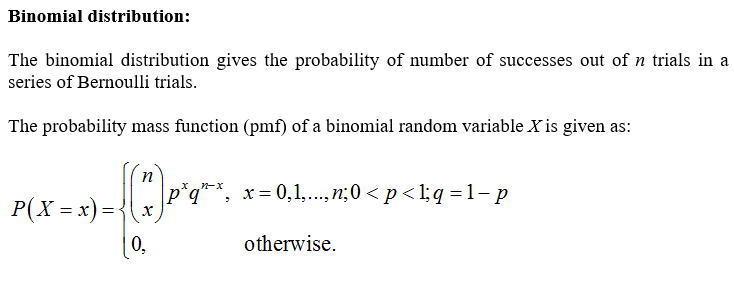# 7.8Topic 8: The Binomial Distribution63. Suppose X follows a Binomial distribution. Is X continuous or discrete? Explain in your ownwords.64. A particular test has a 72% pass rate. A teacher decides to grade tests until 15 people havepassed before stopping for a break. Let X = the number of tests graded before taking a break.Determine if X is binomial by stating and checking all four requirements. If X is binomial,summarize the distribution of X in shorthand notation.65. Suppose 22% of students at a certain university are out of state students. A professor takes arandom sample of 50 students. Let X = the number of out of state students in the sample.Determine if X is binomial by stating and checking all four requirements. If X is binomial,summarize the distribution of X in shorthand notation.66. Sickle-cell disease is a group of inherited blood disorders that can cause pain, anemia, swelling,infections, and stroke. It affects about 0.3% of all Americans. Let X = the number of childrenin a family of 4 that have sickle cell disease. Determine if X is binomial by stating and checkingall four requirements. If X is binomial, summarize the distribution of X in shorthand notation.67. About 10% of the general population is left handed. However, about 25% of baseball players areleft handed.(a) Let Xpopulation. State n and p. Find the expected number of left handers and the standardthe number of left handers in a random sample of 25 people from the generaldeviation.(b) Let X = the number of left handers in a random sample of 25 baseball players. State nand p. Find the expected number of left handers and the standard deviation.68. Suppose 22% of students at a certain university are out of state students. A professor takes arandom sample of 50 students. Let X the number of out of state students in the sample.(a) How many students are expected to be out of state? By how much does this vary?(b) What is the probability that 10 will be out of state?(c) What is the probability that 10 or fewer will be out of state?(d) What is the probability that fewer than 10 will be out of state?69. About 10% of the general population is left handed. However, about 25% of baseball players areleft handed. Suppose we take a random sample of 30 baseball players and let X = the numberof left handed players in the sample.(a) What is the probability that at most 8 are left handed?(b) What is the probability that 8 are left handed?(c) What is the probability that more than 12 are left handed?(d) What is the probability that at least 12 are left handed?

Question
8 views

Question 65

check_circle

Step 1...

### Want to see the full answer?

See Solution

#### Want to see this answer and more?

Solutions are written by subject experts who are available 24/7. Questions are typically answered within 1 hour.*

See Solution
*Response times may vary by subject and question.
Tagged in